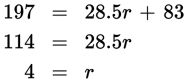# SAT Math Multiple Choice Question 396: Answer and Explanation

### Test Information

Question: 396

6. A botanist collects and models some data and is able to determine that the number of germinated seeds of a certain plant is linearly correlated to the amount of rainfall during the previous month, according to the equation s = 28.5r + 83. In this equation, s is the number of seeds germinated, and r is the amount of rainfall in inches. In a certain geographic region that the botanist is studying, 197 seeds germinated. Approximately how many inches of rainfall did that area receive during the previous month?

• A. 3.1
• B. 4
• C. 7
• D. 9.8

Explanation:

B

Difficulty: Easy

Category: Heart of Algebra / Linear Equations

Strategic Advice: Don't let all the science confuse you. The way in which the variables are defined tells you exactly what to do.

Getting to the Answer: All you need to do is substitute 197 (number of seeds) for s and solve for r (rainfall) using inverse operations.